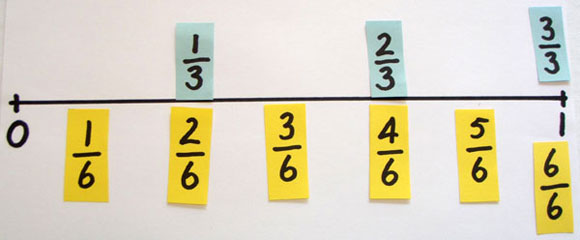Home > Fractions > Good teaching > Equivalence > Linear models

# Linear models

In linear models of fractions, the length of the whole is divided into equal lengths.

A fraction is identified as being a particular distance from the 'start' of the whole.

Dividing strips of paper into equal lengths is an example of using a linear model to represent fractions.

Two or more of these strips placed side-by-side allows comparison of different fractions and identification of equivalent fractions. For example, it could be observed that half a strip is the same length as two-quarters of a strip.

Placing fractions on a number line uses a similar approach, but highlights the fact that some fractions occupy the same position on a number line and therefore represent the same quantity.Visualising equivalent fractions.

## Fraction strips

Students fold strips of paper to create fractions then use the strips to build a fraction wall. The fraction wall facilitates comparison of fractions and the identification of equivalent fractions.

## Fraction wall game

Students use dice to generate fractions that are coloured on an unlabelled fraction wall. Strategies develop for colouring equivalent fractions to fill the fraction wall.